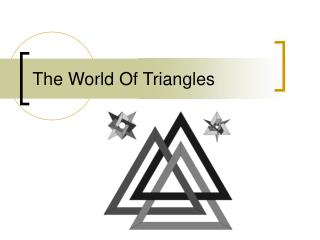DownloadDownload PresentationThe World Of Triangles

# The World Of Triangles

Download Presentation## The World Of Triangles

- - - - - - - - - - - - - - - - - - - - - - - - - - - E N D - - - - - - - - - - - - - - - - - - - - - - - - - - -
##### Presentation Transcript

1. The World Of Triangles

2. Triangles • A triangle is a 3-sided polygon. Every triangle has three sides and three angles. When added together, the three angles equal 180°.

3. Acute Triangles • A triangle in which all three angles are less than 90°.

4. Obtuse Triangles • An obtuse triangle is a triangle in which one of the angles is greater than 90°.

5. Right Triangles • A right triangle is triangle with an angle of 90°.

6. Scalene Triangles • A triangle with three unequal sides.

7. Isosceles Triangles • An isosceles triangle is a triangle with (at least) two equal sides.

8. Isosceles Triangle Theorem • The following movie shows that if two sides of a triangle are congruent, then the angles opposite those sides are congruent.

9. Equilateral Triangles • An equilateral triangle is a triangle with all three sides of equal length.

10. Equiangular Triangles • An equiangular triangle is a triangle which has three equal angles.

11. Congruent Triangles • Congruent triangles are triangles whose corresponding angles and sides are congruent.  • They are exactly the same size and shape.

12. The Hypotenuse • The hypotenuse of a right triangle is the triangle's longest side, i.e., the side opposite the right angle.

13. The Pythagorean Theorem • For a right triangle with legs a and b and hypotenuse c, a2+b2=c2.

14. Triangle Quiz • Please complete the following quiz that covers the information that was just presented to you.

15. If a triangle has three unequal sides it is a ______ triangle. A) Scalene B) Obtuse C) Isosceles Answer: A) Scalene Question 1

16. A triangle which has three equal angles is a ______ triangle. A) Acute B) Equilateral C) Equiangular Answer: C) Equiangular Question 2

17. A triangle with (at least) two equal sides is a ______ triangle. A) Scalene B) Isosceles C) Congruent Answer: B) Isosceles Question 3

18. Two triangles that are equal are called ______ triangles A) Acute B) Obtuse C) Congruent Answer: C) Congruent Question 4

19. A triangle with all three sides of equal length is a ______ triangle. A) Equilateral B) Right C) Equiangular Answer: A) Equilateral Question 5

20. A triangle with an angle of 90° is a ______ triangle. A) Acute B) Right C) Congruent Answer: B) Right Question 6

21. A triangle in which all three angles are less than 90° is a ______ triangle. A) Right B) Acute C) Scalene Answer: B) Acute Question 7

22. A triangle in which one of the angles is greater than 90° is a ______ triangle. A) Obtuse B) Isosceles C) Acute Answer: A) Obtuse Question 8

23. What is the Hypotenuse of a right triangle? A) The smallest angle. B) The side opposite the right angle. C) The right angle. Answer: B) The side opposite the right angle. Question 9

24. The Pythagorean Theorem states … A) pr2 B) (a+b)2/2=c C) a2+b2=c2 Answer: C) a2+b2=c2 Question 10

25. Use the Pythagorean Theorem to solve the following: a = 5 b = 12 c = ? Answer: c = 13 Question 11

26. This powerpoint was kindly donated to www.worldofteaching.com http://www.worldofteaching.com is home to over a thousand powerpoints submitted by teachers. This is a completely free site and requires no registration. Please visit and I hope it will help in your teaching.